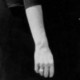# Scala function returns Unit but should return Int

I just started learning Scala last week. I created a stub method in one of my programs but was getting an error. Here’s my function:

``````def howMuch(max: Int) {
var n = 0
n
}``````

I tried to use the result in expression, e.g.

``````var m = 1
var n = howMuch(100)
m += n``````

``````error: overloaded method value - with alternatives:
(x: Int)Int <and>
(x: Char)Int <and>
(x: Short)Int <and>
(x: Byte)Int
cannot be applied to (Unit)
m += n``````

It seemed clear to me that my function should be returning an Int, namely zero. Why was it returning the Unit value?

``````def howMuch(max: Int) = {
1.item says: